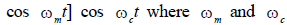# Test: Communication System- 3

## 25 Questions MCQ Test Basic Electronics Engineering for SSC JE (Technical) | Test: Communication System- 3

Description
Attempt Test: Communication System- 3 | 25 questions in 50 minutes | Mock test for Electrical Engineering (EE) preparation | Free important questions MCQ to study Basic Electronics Engineering for SSC JE (Technical) for Electrical Engineering (EE) Exam | Download free PDF with solutions
QUESTION: 1

Solution:
QUESTION: 2

Solution:
QUESTION: 3

### The power spectral density increases as frequency decreases for

Solution:
QUESTION: 4

The spectral density of white noise

Solution:
QUESTION: 5

Which one of the following types of noise gains importance at high frequency

Solution:
QUESTION: 6

For a message signal m(t) = cos (2πfmt) and carrier of frequency fc, which of the following represents a single sideband (SSB) signal

Solution:
QUESTION: 7

Which one of the following is represented by v(t) = 5[cos (106 π t) – sin (103 π t) × sin(106 π t)]

Solution:
QUESTION: 8

The bandwidth requirement of AM wave is

Solution:
QUESTION: 9

Which of the following modulated signals can be detected by an envelope detector

Solution:
QUESTION: 10

Which of the following desirable features are possessed by SSB in comparison to AM

1. Less power is required

2. Bandwidth required is less

3. Antenna size can be reduced

4. Low frequency can be used

Select the correct answer using the codes given below:

Solution:
QUESTION: 11

One of the advantage of base modulation over collector modulation of a transistor Class C amplifier is

Solution:
QUESTION: 12

A modulated signal is given by,

s(t) = m1(t) cos (2 π fct) + m2(t) sin (2 π fct)

where the baseband signal m1(t) and m2(t) have bandwidths of 10 kHz and 15 kHz respectively. The bandwidth of the modulated signal, in kHz, is

Solution:
QUESTION: 13

In a low level modulation AM system, the amplifiers following the modulated stage can be

Solution:
QUESTION: 14

In a 100% amplitude modulated signal, if the total transmitted power is P, then carrier power will be

Solution:
QUESTION: 15

In AM, the percentage of total power in sideband is

Solution:
QUESTION: 16

In an amplitude modulated system, if the total power is 600 W and the power in carrier is 400 W, then the modulation index is

Solution:
QUESTION: 17

The modulation index of an AM is changed from 0 to 1. How does the transmitted power change

Solution:
QUESTION: 18

A 10 kW carrier is sinusoidally modulated by two carriers corresponding to a modulation index of 30% and 40% respectively. The total radiated power is

Solution:
QUESTION: 19

In an AM signal when the modulation index is one, the maximum power Pt (where Pc is the carrier power) is equal to

Solution:
QUESTION: 20

An amplitude modulated signal is [A + 0.5 A] cos wct where wm and wc are respectively, modulating and carrier frequencies. The power efficiency is

Solution:
QUESTION: 21

The maximum power efficiency of an AM modulator is

Solution:
QUESTION: 22

A carrier is amplitude modulated by 4 signals of frequency 10 kHz, 15 kHz, 20 kHz and 25 kHz. What is the bandwidth of the modulated signal

Solution:
QUESTION: 23

VSB modulation as compared to SSB modulation, for band occupies

Solution:
QUESTION: 24

Which of the following analog modulation scheme requires the minimum transmitted power and minimum channel bandwidth

Solution:
QUESTION: 25

In frequency modulation

Solution:Use Code STAYHOME200 and get INR 200 additional OFF Use Coupon Code